# Laboratory #7: Solving partial differential equations using an explicit, finite difference method.¶

Lin Yang & Susan Allen & Carmen Guo

## List of Problems¶

• Problem 1: Numerical solution on a staggered grid.
• Problem 2: Stability of the difference scheme
• Problem 3: Dispersion relation for grid 2
• Problem 4: Choosing most accurate grid
• Problem 5: Numerical solution for no y variation
• Problem 6: Stability on the 2-dimensional grids
• Problem 7: Finite difference form of equations
• Problem 8: Dispersion relation for D-grid
• Problem 9: Accuracy of the approximation on various grids

# 1. Objectives¶

## 1.1 Sections 3 through 6¶

When you have completed these sections you will be able to:

• find the dispersion relation for a set of differential equations (the “real” dispersion relation).

• find the dispersion relation for a set of difference equations (the numerical dispersion relation).

• describe a leap-frog scheme

• construct a predictor-corrector method

• use the given differential equations to determine unspecified boundary conditions as necessary

• describe a staggered grid

• state one reason why staggered grids are used

• explain the physical principle behind the CFL condition

• find the CFL condition for a linear, explicit, numerical scheme

• state one criteria that should be considered when choosing a grid

## 1.2 Section 7¶

TBA

These are the suggested readings for this lab. For more details about the books and papers, click on the reference link.

In :
from IPython.display import Image
import IPython.display as display
# import plotting package and numerical python package for use in examples later
import matplotlib.pyplot as plt
# make the plots happen inline
% matplotlib inline
# import the numpy array handling library
import numpy as np
# import the quiz script
from numlabs.lab7 import quiz7 as quiz
# import the pde solver for a simple 1-d tank of water with a drop of rain
from numlabs.lab7 import rain
# import the dispersion code plotter
from numlabs.lab7 import accuracy2d
# import the 2-dimensional drop solver
from numlabs.lab7 import interactive1
# import the 2-dimensional dispersion relation plotter
from numlabs.lab7 import dispersion_2d


# 3. Physical Example, Poincaré Waves¶

One of the obvious examples of a physical phenomena governed by a partial differential equation is waves. Consider a shallow layer of water and the waves on the surface of that layer. If the depth of the water is much smaller than the wavelength of the waves, the velocity of the water will be the same throughout the depth. So then we can describe the state of the water by three variables: $u(x,y,t)$, the east-west velocity of the water, $v(x,y,t)$, the north-south velocity of the water and $h(x,y,t)$, the height the surface of the water is deflected. As specified, each of these variables are functions of the horizontal position, $(x,y)$ and time $t$ but, under the assumption of shallow water, not a function of $z$.

In oceanographic and atmospheric problems, the effect of the earth’s rotation is often important. We will first introduce the governing equations including the Coriolis force (Full Equations). However, most of the numerical concepts can be considered without all the complications in these equations. We will also consider two simplier sets; one where we assume there is no variation of the variables in the y-direction (No variation in y) and one where, in addition, we assume that the Coriolis force is negligible (Simple Equations).

The solution of the equations including the Coriolis force are Poincaré waves whereas without the Coriolis force, the resulting waves are called shallow water gravity waves.

The remainder of this section will present the equations and discuss the dispersion relation for the two simplier sets of equations. If your wave theory is rusty, consider reading Appendix A in Cushman-Roisin, 1994.

### Full Equations¶

The linear shallow water equations on an f-plane over a flat bottom are

(Full Equations, Eqn 1) $$\frac{\partial u}{\partial t} - fv = -g\frac{\partial h}{\partial x}$$
(Full Equations, Eqn 2) $$\frac{\partial v}{\partial t} + fu = -g\frac{\partial h}{\partial y}$$
(Full Equations, Eqn 3) $$\frac{\partial h}{\partial t} + H\frac{\partial u}{\partial x} + H\frac{\partial v}{\partial y} = 0$$
where

• $\vec{u} = (u,v)$ is the horizontal velocity,

• $f$ is the Coriolis frequency,

• $g$ is the acceleration due to gravity,

• $h$ is the surface elevation, and

• $H$ is the undisturbed depth of the fluid.

### No variation in y¶

To simplify the problem assume there is no variation in y. This simplification gives:

(No variation in y, first eqn) $$\frac{\partial u}{\partial t} - fv = -g\frac{\partial h}{\partial x}$$
(No variation in y, second eqn) $$\frac{\partial v}{\partial t} + fu = 0$$
(No variation in y, third eqn) $$\frac{\partial h}{\partial t} + H\frac{\partial u}{\partial x} = 0$$

### Simple Equations¶

If we consider waves in the absence of the earth’s rotation, $f=0$, which implies $v=0$ and we get

$$\frac{\partial u}{\partial t} = -g\frac{\partial h}{\partial x}$$
$$\frac{\partial h}{\partial t} + H\frac{\partial u}{\partial x} = 0$$

These simplified equations give shallow water gravity waves. For example, a solution is a simple sinusoidal wave:

(wave solution- h) $$h = h_{0}\cos{(kx - \omega t)}$$
(wave solution- u) $$u = \frac{h_{0}\omega}{kH}\cos{(kx - \omega t)}$$
where $h_{0}$ is the amplitude, $k$ is the wavenumber and $\omega$ is the frequency (See Cushman-Roisin, 1994 for a nice review of waves in Appendix A).

Substitution of (wave solution- h) and (wave solution- u) back into the differential equations gives a relation between $\omega$ and k. Confirm that

(Analytic Dispersion Relation) $$\omega^2 = gHk^2,$$
which is the dispersion relation for these waves.

### No variation in y¶

Now consider $f\not = 0$.

By assuming $$h= h_{0}e^{i(kx - \omega t)}$$ $$u= u_{0}e^{i(kx - \omega t)}$$ $$v= v_{0}e^{i(kx - \omega t)}$$

and substituting into the differential equations, eg, for (No variation in y, first eqn) $$-iwu_{0}e^{i(kx - \omega t)} - fv_{0}e^{i(kx - \omega t)} + ikgh_{0}e^{i(kx - \omega t)} = 0$$ and cancelling the exponential terms gives 3 homogeneous equations for $u_{0}$, $v_{0}$ and $h_{0}$. If the determinant of the matrix derived from these three equations is non-zero, the only solution is $u_{0} = v_{0} = h_{0} = 0$, NO WAVE! Therefore the determinant must be zero.

### Quiz: Find the Dispersion Relation¶

What is the dispersion relation for 1-dimensional Poincare waves?

A) $\omega^2 = f^2 + gH (k^2 + \ell^2)$

B) $\omega^2 = gH k^2$

C) $\omega^2 = f^2 + gH k^2$

D) $\omega^2 = -f^2 + gH k^2$

In the following, replace 'x' by 'A', 'B', 'C' or 'D' and run the cell.

In :
print (quiz.dispersion_quiz(answer = 'x'))

Acceptable answers are 'A', 'B', 'C' or 'D'


# 4. Numerical Solution¶

### Simple Equations¶

Consider first the simple equations with $f = 0$. In order to solve these equations numerically, we need to discretize in 2 dimensions, one in space and one in time. Consider first the most obvious choice, shown in Figure Unstaggered Grid.

In :
Image(filename='images/nonstagger.png',width='40%')

Out: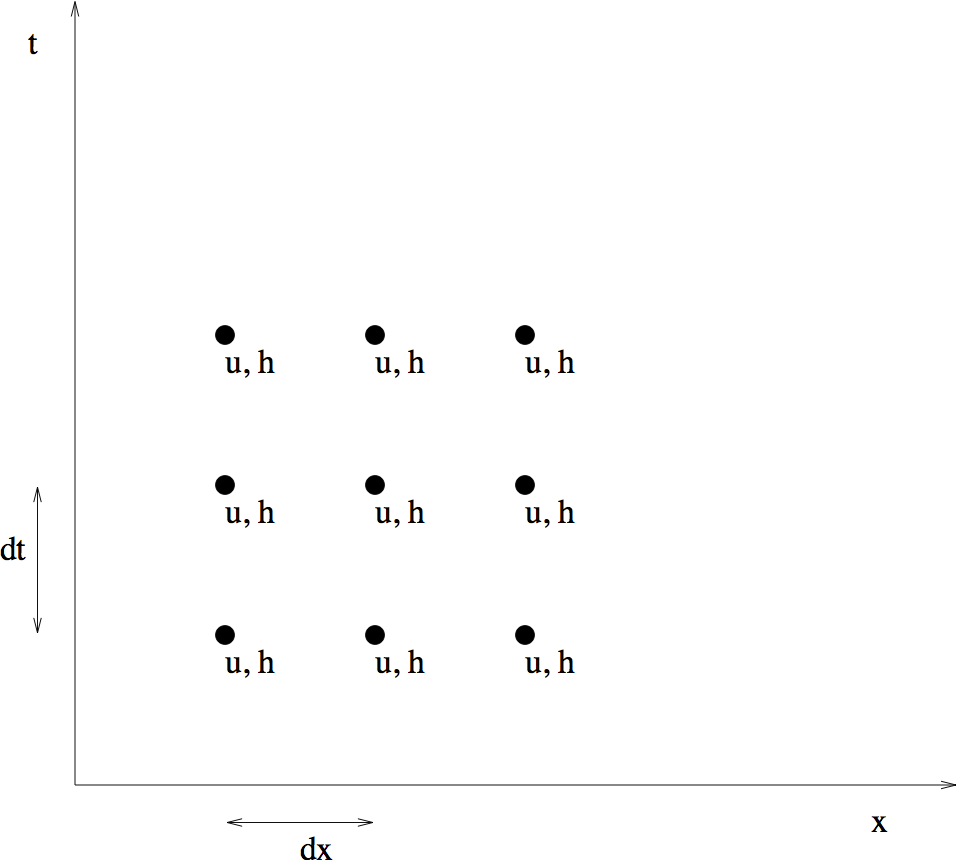**Figure Unstaggered Grid.**

We will use centred difference schemes in both $x$ and $t$. The equations become:

(Non-staggered, Eqn One) $$\frac {u(t+dt, x)-u(t-dt, x)}{2 dt} + g \frac {h(t, x+dx) - h(t, x-dx)}{2dx} = 0$$
(Non-staggered, Eqn Two) $$\frac {h(t+dt, x)-h(t-dt, x)}{2 dt} + H \frac {u(t, x+dx) - u(t, x-dx)}{2dx} = 0$$
We can rearrange these equations to give $u(t+dt, x)$ and $h(t+dt, x)$. For a small number of points, the resulting problem is simple enough to solve in a notebook.

For a specific example, consider a dish, 40 cm long, of water 1 cm deep. Although the numerical code presented later allows you to vary the number of grid points, in the discussion here we will use only 5 spatial points, a distance of 10 cm apart. The lack of spatial resolution means the wave will have a triangular shape. At $t=0$ a large drop of water lands in the centre of the dish. So at $t=0$, all points have zero velocity and zero elevation except at $x=3dx$, where we have $$h(0, 3dx) = h_{0} = 0.01 cm$$

A centred difference scheme in time, such as defined by equations (Non-staggered, Eqn One) and (Non-staggered, Eqn Two), is usually refered to as a Leap frog scheme. The new values, $h(t+dt)$ and $u(t+dt)$ are equal to values two time steps back $h(t-dt)$ and $u(t-dt)$ plus a correction based on values calculated one time step back. Hence the time scheme “leap-frogs” ahead. More on the consequences of this process can be found in section 8.4 Computational Mode.

As a leap-frog scheme requires two previous time steps, the given conditions at $t=0$ are not sufficient to solve (Non-staggered, Eqn One) and (Non-staggered, Eqn Two). We need the solutions at two time steps in order to step forward.

## 4.1 Predictor-Corrector to Start¶

In section 4.2.2 of Lab 2, predictor-corrector methods were introduced. We will use a predictor-corrector based on the forward Euler scheme, to find the solution at the first time step, $t=dt$. Then the second order scheme (Non-staggered, Eqn One), (Non-staggered, Eqn Two) can be used.

Using the forward Euler Scheme, the equations become

$$\frac {u(t+dt, x)-u(t, x)}{dt} + g \frac {h(t, x+dx) - h(t, x-dx)}{2dx} = 0$$
$$\frac {h(t+dt, x)-h(t, x)}{dt} + H \frac {u(t, x+dx) - u(t, x-dx)}{2dx} = 0$$
1. Use this scheme to predict $u$ and $h$ at $t=dt$.

2. Average the solution at $t=0$ and that predicted for $t=dt$, to estimate the solution at $t=\frac{1}{2}dt$. You should confirm that this procedure gives: $$u(\frac{dt}{2}) = \left\{ \begin{array}{ll} 0 & { x = 3dx}\\ \left({-gh_{0}dt}\right)/\left({4dx}\right) & { x = 2dx}\\ \left({gh_{0}dt}\right)/\left({4dx}\right) & { x = 4dx} \end{array} \right.$$

$$h(\frac{dt}{2}) = \left\{ \begin{array}{ll} h_{0} & { x = 3dx}\\ 0 & { x \not= 3dx} \end{array} \right.$$

3. The corrector step uses the centred difference scheme in time (the leap-frog scheme) with a time step of ${dt}/{2}$ rather than dt. You should confirm that this procedure gives: $$u(dt) = \left\{ \begin{array}{ll} 0 & { x = 3dx}\\ \left({-gh_{0}dt}\right)/\left({2dx}\right) & { x = 2dx}\\ \left({gh_{0}dt}\right)/\left({2dx}\right) & { x = 4dx} \end{array} \right.$$

$$h(dt) = \left\{ \begin{array}{ll} 0 & { x = 2dx, 4dx}\\ h_{0} - \left({gHdt^2 h_{0}}\right)/\left({4dx^2}\right) & { x = 3dx} \end{array} \right.$$

Note that the values at $x=dx$ and $x=5dx$ have not been specified. These are boundary points and to determine these values we must consider the boundary conditions.

## 4.2 Boundary Conditions¶

If we are considering a dish of water, the boundary conditions at $x=dx, 5dx$ are those of a wall. There must be no flow through the wall. $$u(dx) = 0$$ $$u(5dx) = 0$$ But these two conditions are not sufficient; we also need $h$ at the walls. If $u=0$ at the wall for all time then $\partial u/\partial t=0$ at the wall, so $\partial h/\partial x=0$ at the wall. Using a one-sided difference scheme this gives $$\frac {h(2dx) - h(dx)}{dx} = 0$$ or$$h(dx) = h(2dx)$$ and$$\frac {h(4dx) - h(5dx)}{dx} = 0$$ or$$h(5dx) = h(4dx)$$ which gives the required boundary conditions on $h$ at the wall.

## 4.3 Simple Equations on a Non-staggered Grid¶

1. Given the above equations and boundary conditions, we can find the values of $u$ and $h$ at all 5 points when $t = 0$ and $t = dt$.

2. From (Non-staggered, Eqn One) and (Non-staggered, Eqn Two), we can find the values of $u$ and $h$ for $t = 2dt$ using $u(0, x)$, $u(dt, x)$, $h(0, x)$, and $h(dt, x)$.

3. Then we can find the values of $u$ and $h$ at $t = 3dt$ using $u(dt, x)$, $u(2dt, x)$, $h(dt, x)$, and $h(2dt, x)$.

We can use this approach recursively to determine the values of $u$ and $h$ at any time $t = n * dt$. The python code that solves this problem is provided in the file rain.py. It takes two arguments, the first is the number of time steps and the second is the number of horizontal grid points.

The output is two coloured graphs. The color represents time with black the earliest times and red later times. The upper plot shows the water velocity (u) and the lower plot shows the water surface. To start with the velocity is 0 (black line at zero across the whole domain) and the water surface is up at the mid point and zero at all other points (black line up at midpoint and zero elsewhere)

Not much happens in 6 time-steps. Do try longer and more grid points.

In :
rain.rain([6, 5])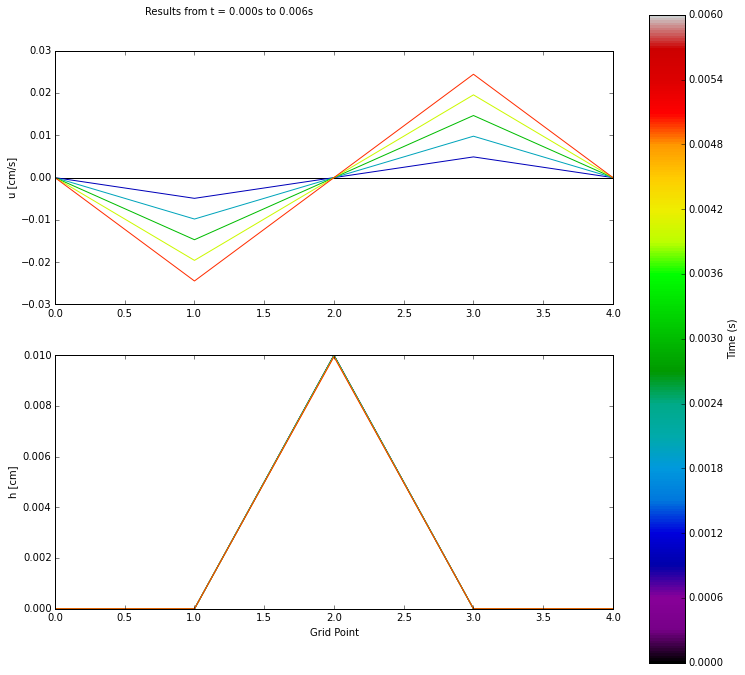If you want to change something in the script (say the colormap I've chosen, spectral, doesn't work for you), you can edit rain.py in an editor or spyder. To make it take effect here though, you have to reload rain. See next cell for how to. You will also need to do this if you do problem one or other tests by changing rain.py but running in a notebook.

In :
import importlib

Out:
<module 'numlabs.lab7.rain' from '/Users/sallen/Documents/numeric/numlabs/lab7/rain.py'>

## 4.4 Staggered Grids¶

After running the program with different numbers of spatial points, you will discover that the values of $u$ are always zero at the odd numbered points, and that the values of $h$ are always zero at the even numbered points. In other words, the values of $u$ and $h$ are zero in every other column starting from $u(t, dx)$ and $h(t, 2dx)$, respectively.

A look at (Non-staggered, Eqn One) and (Non-staggered, Eqn Two) can help us understand why this is the case:

$u(t + dt, x)$ is dependent on $h(t , x + dx)$ and $h(t , x - dx)$,

but $h(t , x + dx)$ is in turn dependent on $u$ at $x + 2dx$ and at $x$,

and $h(t , x - dx)$ is in turn dependent on $u$ at $x - 2dx$ and at $x$.

Thus, if we just look at $u$ at a particular $x$, $u(x)$ will depend on $u(x + 2dx)$, $u(x - 2dx)$, $u(x + 4dx)$, $u(x - 4dx)$, $u(x + 6dx)$, $u(x - 6dx),$ ... but not on $u(x + dx)$ or $u(x - dx)$. Therefore, the problem is actually decoupled and consists of two independent problems: one problem for all the $u$’s at odd numbered points and all the $h$’s at even numbered points, and the other problem for all the $u$’s at even numbered points and all the $h$’s at odd numbered points, as shown in Figure Unstaggered Dependency.

In :
Image(filename='images/dependency.png',width='50%')

Out: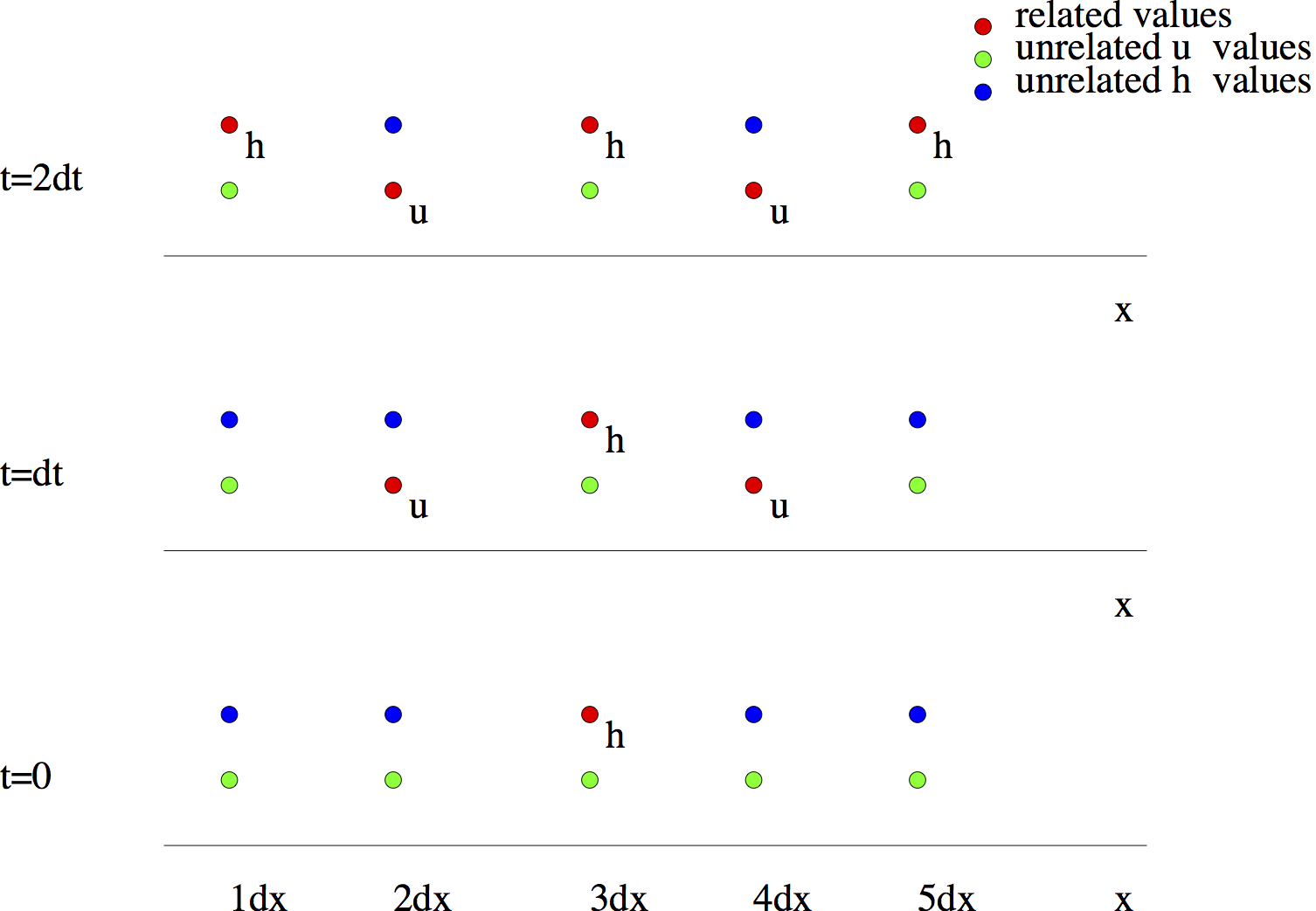**Figure Unstaggered Dependency**

In either problem, only the variable that is relevant to that problem will be considered at each point. So for one problem, if at point $x$ we consider the $u$ variable, at $x + dx$ and $x -dx$ we consider $h$. In the other problem, at the same point $x$, we consider the variable $h$.

Now we can see why every second $u$ point and $h$ point are zero for rain. We start with all of $u(dx), h(2dx), u(3dx), h(4dx), u(5dx) = 0$, which means they remain at zero.

Since the original problem can be decoupled, we can solve for $u$ and $h$ on each decoupled grid separately. But why solve two problems? Instead, we solve for $u$ and $h$ on a single staggered grid; whereas before we solved for $u$ and $h$ on the complete, non-staggered grid. Figure Decoupling shows the decoupling of the grids.

In :
Image(filename='images/decoupling.png',width='50%')

Out: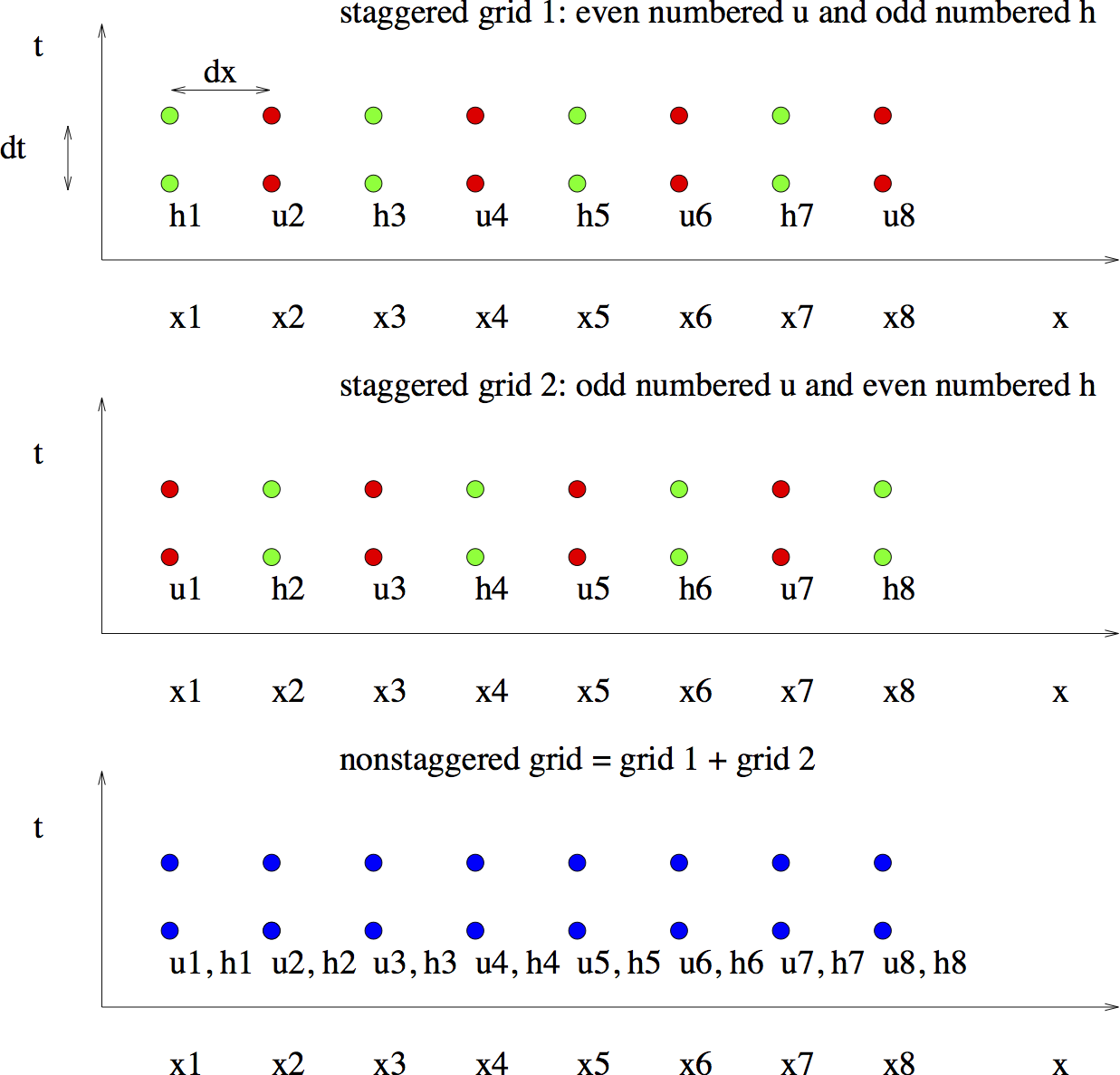**Figure Decoupling**: The two staggered grids and the unstaggered grid. Note that the unstaggered grid has two variables at each grid/time point whereas the staggered grids only have one.

Now consider the solution of the same problem on a staggered grid. The set-up of the problem is slightly different this time; we are considering 4 spatial points in our discussion instead of 5, shown in Figure Staggered Grid. We will also be using $h_{i}$ and $u_{i}$ to denote the spatial points instead of $x = dx * i$.

In :
Image(filename='images/stagger.png',width='50%')

Out: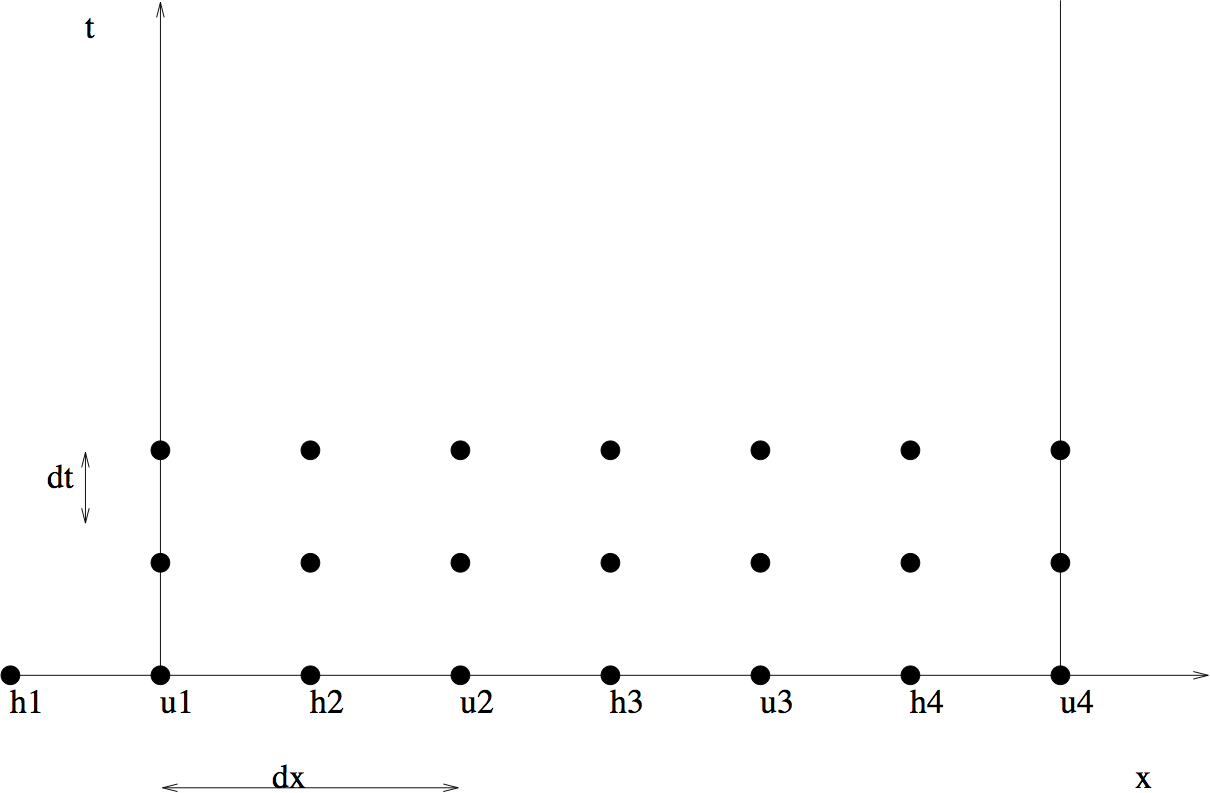**Figure Staggered Grid**: The staggered grid for the drop in the pond problem.

The original equations, boundary and initial conditions are changed to reflect the staggered case. The equations are changed to the following:

(Staggered, Eqn 1) $$\frac {u_{i}(t+dt)-u_{i}(t-dt)}{2 dt} + g \frac {h_{i + 1}(t) - h_{i}(t)}{dx} = 0$$
(Staggered, Eqn 2) $$\frac {h_{i}(t+dt)-h_{i}(t-dt)}{2 dt} + H \frac {u_{i}(t) - u_{i - 1}(t)}{dx} = 0$$

The initial conditions are: At $t = 0$ and $t = dt$, all points have zero elevation except at $h_{3}$, where $$h_{3}(0) = h_{0}$$ $$h_{3}(dt) = h_{3}(0) - h_{0} Hg \frac{dt^2}{dx^2}$$ At $t = 0$ and $t = dt$, all points have zero velocity except at $u_{2}$ and $u_{3}$, where $$u_{2}(dt) = - h_{0} g \frac{dt}{dx}$$ $$u_{3}(dt) = - u_{2}(dt)$$ This time we assume there is a wall at $u_{1}$ and $u_{4}$, so we will ignore the value of $h_{1}$. The boundary conditions are: $$u_{1}(t) = 0$$ $$u_{4}(t) = 0$$

## Problem One¶

Modify rain.py to solve this problem (Simple equations on a staggered grid). Submit your code and a final plot for one case.

# 5. Stability: the CFL condition¶

In the previous problem, $dt = 0.001s$ is used to find $u$ and $h$. If you increase $dt$ by 100 times, and run rain.py on your staggered grid code again, you can see that the magnitude of $u$ has increased from around 0.04 to $10^8$! Try this by changing $dt = 0.001$ to $dt = 0.1$ in the code and compare the values of $u$ when run with different values of $dt$. This tells us that the scheme we have been using so far is unstable for large values of $dt$.

To understand this instability, consider a spoked wagon wheel in an old western movie (or a car wheel with a pattern in a modern TV movie) such as that shown in Figure Wheel.

In :
Image(filename='images/wheel_static.png',width='35%')

Out:**Figure Wheel**: A spoked wagon wheel.

Sometimes the wheels appear to be going backwards. Both TV and movies come in frames and are shown at something like 30 frames a second. So a movie discretizes time. If the wheel moves just a little in the time step between frames, your eye connects the old position with the new position and the wheel moves forward $-$ a single frame is shown in Figure Wheel Left.

In :
Image(filename='images/wheel_left.png',width='35%')

Out: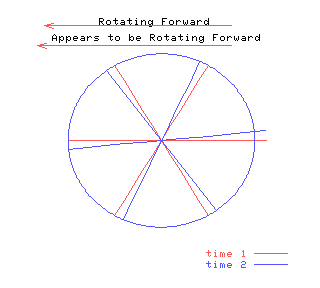**Figure Wheel Left:** The wheel appears to rotate counter-clockwise if its speed is slow enough.
In :
vid = display.YouTubeVideo("hgQ66frbBEs", modestbranding=1, rel=0, width=500)
display.display(vid)


However, if the wheel is moving faster, your eye connects each spoke with the next spoke and the wheel seems to move backwards $-$ a single frame is depicted in Figure Wheel Right.

In :
Image(filename='images/wheel_right.png',width='35%')

Out: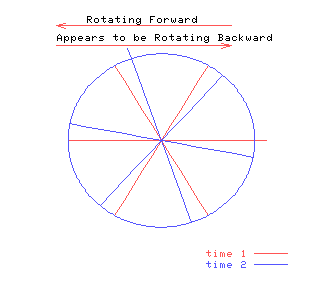**Figure Wheel Right**: When the wheel spins quickly enough, it appears to rotate clockwise!
In :
vid = display.YouTubeVideo("w8iQIwX-ek8", modestbranding=1, rel=0, width=500)
display.display(vid)


In a similar manner, the time discretization of any wave must be small enough that a given crest moves less than half a grid point in a time step. Consider the wave pictured in Figure Wave.

In :
Image(filename='images/wave_static.png',width='65%')

Out: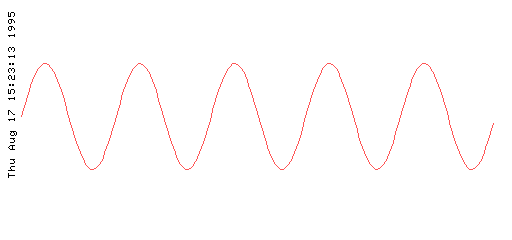**Figure Wave**: A single frame of the wave.

If the wave moves slowly, it seems to move in the correct direction (i.e. to the left), as shown in Figure Wave Left.

In :
Image(filename='images/wave_left.png',width='65%')

Out: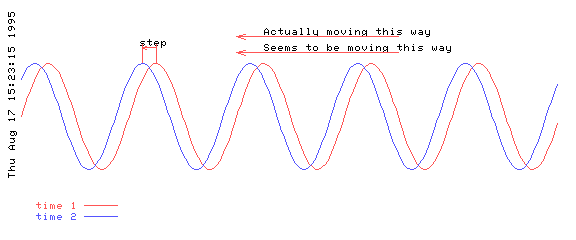**Figure Wave Left**: The wave moving to the left also appears to be moving to the left if it’s speed is slow enough.
In :
vid = display.YouTubeVideo("CVybMbfYRXM", modestbranding=1, rel=0, width=500)
display.display(vid)


However if the wave moves quickly, it seems to move backward, as in Figure Wave Right.

In :
Image(filename='images/wave_right.png',width='65%')

Out: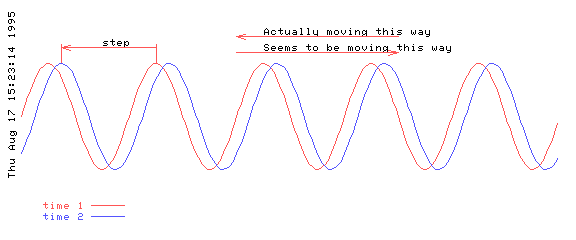Figure Wave Right: If the wave moves too rapidly, then it appears to be moving in the opposite direction.

In :
vid = display.YouTubeVideo("al2VrnkYyD0", modestbranding=1, rel=0, width=500)
display.display(vid)


In summary, an explicit numerical scheme is unstable if the time step is too large. Such a large time step does not resolve the process being modelled. Now we need to calculate, for our problem, the maximum value of the time step is for which the scheme remains stable. To do this calculation we will derive the dispersion relation of the waves in the numerical scheme. The maximum time step for stability will be the maximum time step for which all waves either maintain their magnitude or decay.

Mathematically, consider the equations (Staggered, Eqn 1) and (Staggered, Eqn 2). Let $x=md$ and $t=p\, dt$ and consider a solution

(u-solution) \begin{aligned} u_{mp} &=& {\cal R}e \{ {\cal U} \exp [i(kx - \omega t)] \}\\ &=& {\cal R}e \{ {\cal U} \exp [i(kmd - \omega p\, dt)] \} \nonumber\end{aligned} \begin{aligned} h_{mp} &=& {\cal R}e \{ {\cal H} \exp [i(k[x - dx/2] - \omega t)] \}\\ &=& {\cal R}e \{ {\cal H} \exp [i(k[m - 1/2]d - \omega p\, dt)] \} \nonumber\end{aligned} where ${\cal R}e$ means take the real part and ${\cal U}$ and ${\cal H}$ are constants. Substitution into ([Staggered, Eqn 1](#lab7:eq:staggerGrid1)) and ([Staggered, Eqn 2](#lab7:eq:staggerGrid2)) gives two algebraic equations in ${\cal U}$ and ${\cal H}$ which can be written: $$\left[ \begin{array}{cc} - \sin(\omega dt)/ dt & 2 g \sin(kd/2)/d \\ 2 H \sin(kd/2)/d & -\sin(\omega \, dt)/ dt \\ \end{array} \right] \left[ \begin{array}{c} {\cal U}\\ {\cal H}\\ \end{array} \right] = 0.$$
where $\exp(ikd)-\exp(-ikd)$ has been written $2 i \sin(kd)$ etc. In order for there to be a non-trivial solution, the determinant of the matrix must be zero. This determinant gives the dispersion relation
(Numerical Dispersion Relation) $$\frac{\sin^2(\omega \, dt)}{dt^2} = 4 gH \frac {\sin^2(kd/2)}{d^2}$$
Which can be compared to the ([Analytic Dispersion Relation](#lab7:eq:disp)), the “real” dispersion relation. In particular, if we decrease both the time step and the space step, $dt \rightarrow 0$ and $d \rightarrow 0$, ([Numerical Dispersion Relation](#lab7:eq:numerDisp)) approaches ([Analytic Dispersion Relation](#lab7:eq:disp)). The effect of just the discretization in space can be found by letting just $dt \rightarrow 0$ which gives
(Continuous Time, Discretized Space Dispersion Relation) $$\omega^2 = 4 gH \frac {\sin^2(kd/2)}{d^2}$$

The “real” dispersion relation, (Analytic Dispersion Relation), and the numerical dispersion relation with continuous time, (Continuous Time, Discretized Space Dispersion Relation), both give $\omega^2$ positive and therefore $\omega$ real. However, this is not necessarily true for the numerical dispersion relation (Numerical Dispersion Relation). What does a complex $\omega$ mean? Well, go back to (u-solution). A complex $\omega = a + ib$ gives $u \propto \exp(-iat)\exp(bt)$. The first exponential is oscillatory (like a wave) but the second gives exponential growth if $b$ is positive or exponential decay if $b$ is negative. Obviously, for a stable solution we must have $b \le 0$. So, using (Numerical Dispersion Relation) we must find $\omega$ and determine if it is real.

Now, because (Numerical Dispersion Relation) is a transcendental equation, how to determine $\omega$ is not obvious. The following works:

• Re-expand $\sin(\omega\,dt)$ as $(\exp(i \omega\,dt)-\exp(-i\omega\,dt))/2i$.

• Write $\exp(-i\omega\,dt)$ as $\lambda$ and note that this implies $\exp(i\omega\, dt) = 1/\lambda$. If $\omega dt = a + ib$ then $b = ln |\lambda|$. For stability the magnitude of $\lambda$ must be less than one.

• Write $4 gH \sin^2(kd/2)/d^2$ as $q^2$, for brevity.

• Substitute in (Numerical Dispersion Relation) which gives: $$-(\lambda-1/\lambda)^2 = 4 q^2 dt^2$$ or $$\lambda^4 - 2 (1 - 2 q^2 dt^2) \lambda^2 + 1 = 0$$ or

(Lambda Eqn) $$\lambda = \pm \left(1-2q^2 dt^2 \pm 2 q dt \left( q^2 dt^2 - 1 \right)^{1/2} \right)^{1/2}$$

A plot of the four roots for $\lambda$ is shown below in Figure Roots.

In :
Image(filename='images/allmag.png',width='45%')

Out: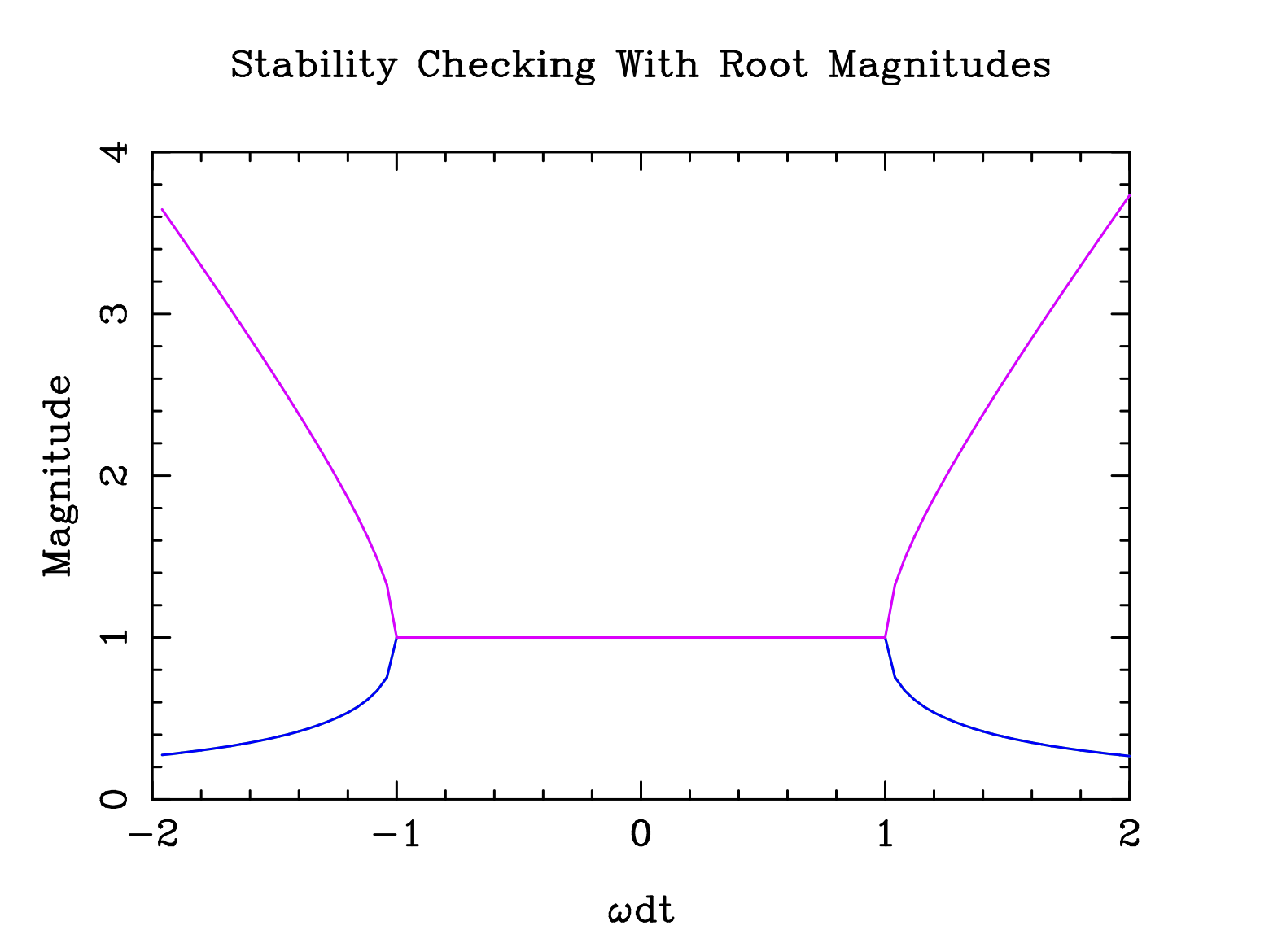**Figure Roots**: Magnitude of the four roots of $\lambda$ as a function of $q dt$ (not $\omega dt$).

The four roots correspond to the “real” waves travelling to the right and left, as well two computational modes (see Section 8.4 Computational Mode for more information). The plots for the four roots overlap, so it is most helpful to view separate plots for each of the roots.

In :
Image(filename='images/multimag.png',width='60%')

Out: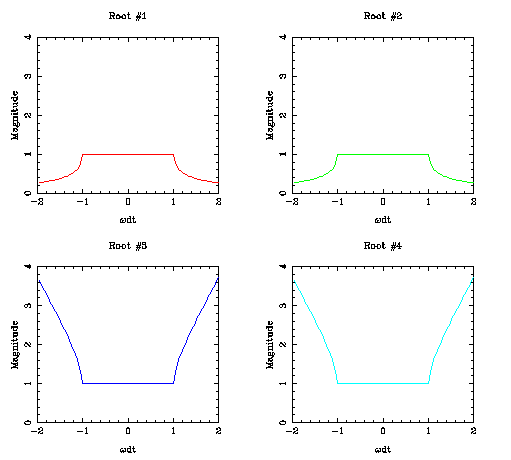**Figure Separate Roots**: Magnitude of the four roots of $\lambda$ as a function of $q dt$ (not $\omega dt$).

Now for stability $\lambda$ must have a magnitude less than or equal to one. From Figure Roots, it is easy to see that this is the same as requiring that $|q dt|$ be less than 1.0.

Substituting for $q$ $$1 > q^2 dt^2 = \frac {4gH}{d^2} \sin^2(kd/2) dt^2$$ for all $k$.

The maximum wavenumber that can be resolved by a grid of size $d$ is $k = \pi/d$. At this wavenumber, the sine takes its maximum value of 1. So the time step

$$dt^2 < \frac { d^2}{4 g H}$$

For this case ($f = 0$) the ratio of the space step to the time step must be greater than the wave speed $\sqrt {gH}$, or $$d / dt > 2 \sqrt{gH}.$$ This stability condition is known as the CFL condition (named after Courant, Friedrich and Levy).

On a historical note, the first attempts at weather prediction were organized by Richardson using a room full of human calculators. Each person was responsible for one grid point and passed their values to neighbouring grid points. The exercise failed dismally, and until the theory of CFL, the exact reason was unknown. The equations Richardson used included fast sound waves, so the CFL condition was $$d/dt > 2 \times 300 {\rm m/s}.$$ Richardson’s spatial step, $d$, was too small compared to $dt$ and the problem was unstable.

## Problem Two¶

a) Find the CFL condition (in seconds) for $dt$ for the Python example in Problem One. Test your value.

b) Find the CFL condition (in seconds) for $dt$ for the Python example in rain.py, ie., for the non-staggered grid. Test your value.

# 6. Accuracy¶

A strong method to determine the accuracy of a scheme is to compare the numerical solution to an analytic solution. The equations we are considering are wave equations and so we will compare the properties of the waves. Wave properties are determined by the dispersion relation and we will compare the numerical dispersion relation and the exact, continuous analytic dispersion relation. Both the time step and the space step (and as we’ll see below the grid) affect the accuracy. Here we will only consider the effect of the space step. So, consider the numerical dispersion relation assuming $dt \rightarrow 0$ (reproduced here from (Continuous Time, Discretized Space Dispersion Relation)) $$\omega^2 = 4 gH \frac {\sin^2(kd/2)}{d^2}$$ with the exact, continuous dispersion relation (Analytic Dispersion Relation) $$\omega^2 = gHk^2$$

We can plot the two dispersion relations as functions of $kd$, The graph is shown in Figure Simple Accuracy.

In :
Image(filename='images/simple_accuracy.png',width='50%')

Out: